Next: The PN Diode as Up: The PN Junction and Previous: The PN Junction and

## Current in the Diode

The behaviour of a diode depends on its polarity in the circuit (figure 4.2). If the diode is reverse biased (positive potential on N-type material) the depletion region increases. The only charge carriers able to support a net current across the PN junction are the minority carriers and hence the reverse current is very small. A forward-biased diode (positive potential on P-type material) has a decreased depletion region; the majority carriers can diffuse across the junction. The voltage may become high enough to eliminate the depletion region entirely.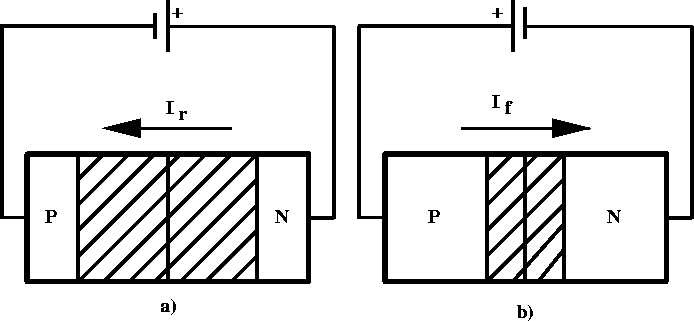Figure 4.2:  Diode circuit connections: a) reversed biased and b) forward biased.

An approximation to the current in the PN junction region is given by (shown in figure 4.3a)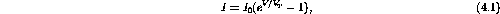where both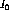and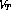are temperature dependent. This equation gives a reasonably accurate prediction of the current-voltage relationship of the PN junction itself - especially the temperature variation - and can be improved somewhat by choosingandempirically to fit a particular diode. However, for a real diode, other factors are also important: in particular, edge effects around the border of the junction cause the actual reverse current to increase slightly with reverse voltage, and the finite conductivity of the doped semi-conductor ultimately restricts the forward current to a linear increase with increasing applied voltage. A better current-voltage curve for the real diode is shown in the figure 4.3b.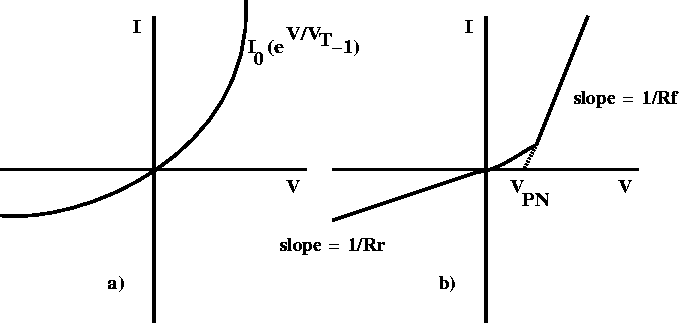Figure 4.3:  Current versus voltage a) in the PN junction region and b) for an actual PN diode.

Various regions of the curve can be identified: the linear region of forward-biasing, a non-linear transition region, a turn-on voltage (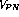) and a reverse-biased region. We can assign a dynamic resistance to the diode in each of the linear regions: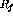in the forward-biased region and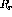in the reverse-biased region. These resistances are defined as the inverse slope of the curve: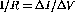. The voltage, represents the effective voltage drop across a forward-biased PN junction (the turn-on voltage). For a germanium diode,is approximately 0.3 V, while for a silicon diode it is close to 0.6 V.Next: The PN Diode as Up: The PN Junction and Previous: The PN Junction and

Doug Gingrich
Tue Jul 13 16:55:15 EDT 1999